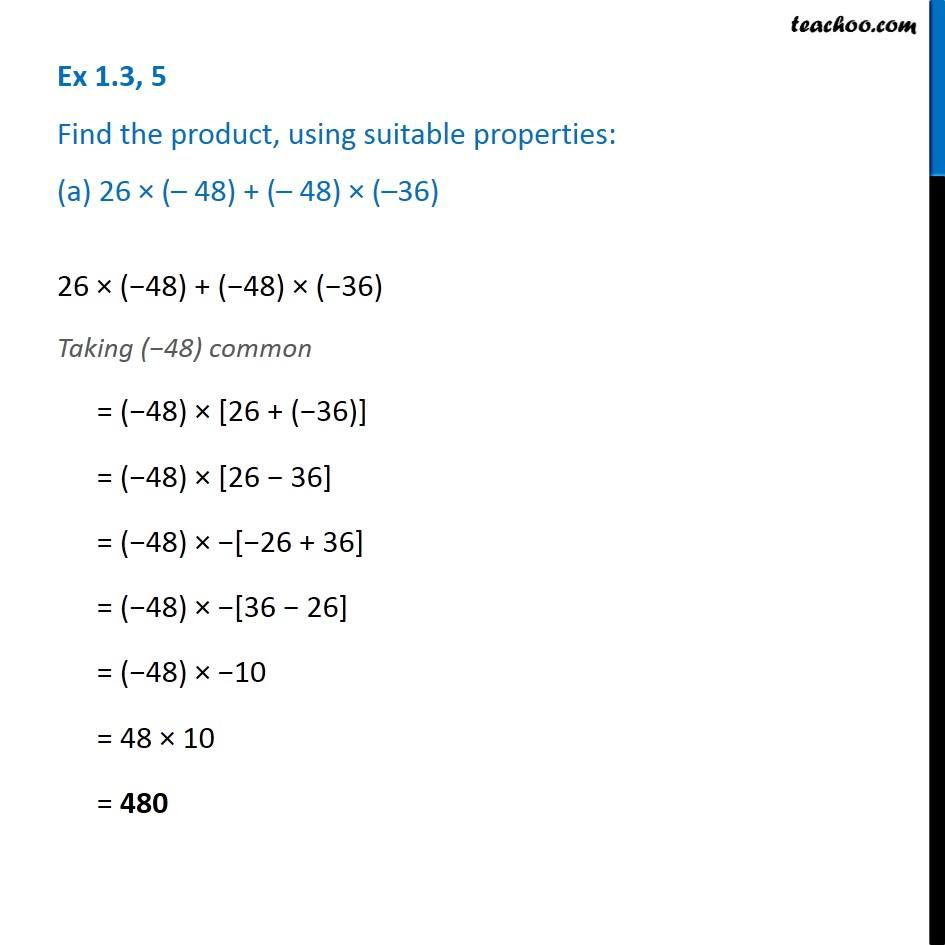1. Chapter 1 Class 7 Integers
2. Concept wise
3. Distributive law in Integers

Transcript

Ex 1.3, 5 Find the product, using suitable properties: (a) 26 × (– 48) + (– 48) × (–36) 26 × (−48) + (−48) × (−36) Taking (−48) common = (−48) × [26 + (−36)] = (−48) × [26 − 36] = (−48) × −[−26 + 36] = (−48) × −[36 − 26] = (−48) × −10 = 48 × 10 = 480